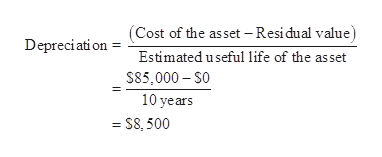# A Kubota tractor acquired on January 8 at a cost of \$85,000 has an estimated useful life of 10 years. Assuming that it will have no residual value, determine the depreciation for each of the first two years (a) by the straight-line method and (b) by the double-decliningbalance method.

Question
2 views

A Kubota tractor acquired on January 8 at a cost of \$85,000 has an estimated useful life of 10 years. Assuming that it will have no residual value, determine the depreciation for each of the first two years (a) by the straight-line method and (b) by the double-decliningbalance method.

check_circle

Step 1

Straight-line Depreciation: Under the straight-line method of depreciation, the same amount of depreciation is allocated every year over the estimated useful life of an asset. The formula to calculate the depreciation cost of the asset using the residual value is shown as below:

Step 2

(a)

Determine the amount of depreciation for the first year.

Cost of the refrigerator= \$85,000

Estimated Useful Life =10 yearshelp_outlineImage Transcriptionclose(Cost of the asset – Residual value) Depreciati on Estimated useful life of the asset S85,000 – So 10 years = S8, 500 fullscreen
Step 3

Determine the amount of depreciation for the second year.

Under the straight-line method, the amount of depreciation in each year remains same....

### Want to see the full answer?

See Solution

#### Want to see this answer and more?

Solutions are written by subject experts who are available 24/7. Questions are typically answered within 1 hour.*

See Solution
*Response times may vary by subject and question.
Tagged in